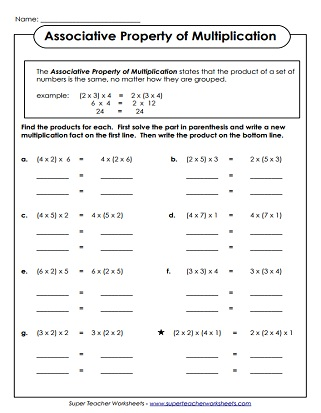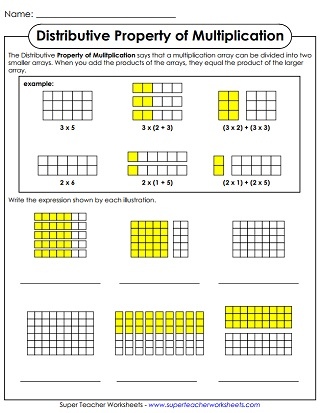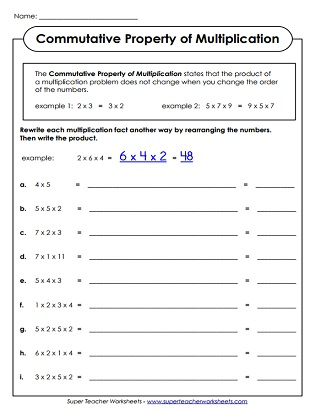# Properties of Multiplication

Printable worksheets for reviewing the associative, distributive, commutative, and identity properties of multiplication.## Distributive Property

This graphical worksheet has arrays to help students visualize how the distributive property of multiplication works.
In this worksheet, students will use the distributive property of multiplication solve basic problems.

## Associative Property

According to the associative property of multiplication, it doesn't matter how the numbers in a given multiplication problem are grouped. In this worksheet, students practice grouping problems in different ways. Each problem has 3 numbers.

## Commutative Property

According to the commutative property of multiplication, numbers in a multiplication problem can be in any order. In this worksheet, students rearrange the numbers in each multiplication problem, then solve.

Basic Multiplication Worksheets

Learn and practice basic facts up to 10 or 12 with these printable games, lessons, and worksheets.

Multi-Digit Multiplication Worksheets

These worksheets have 2, 3, and 4-digit multiplication problems.

These worksheets focus on the associative & commutative properties of addition.

## Worksheet ImagesMy Account
Site Information